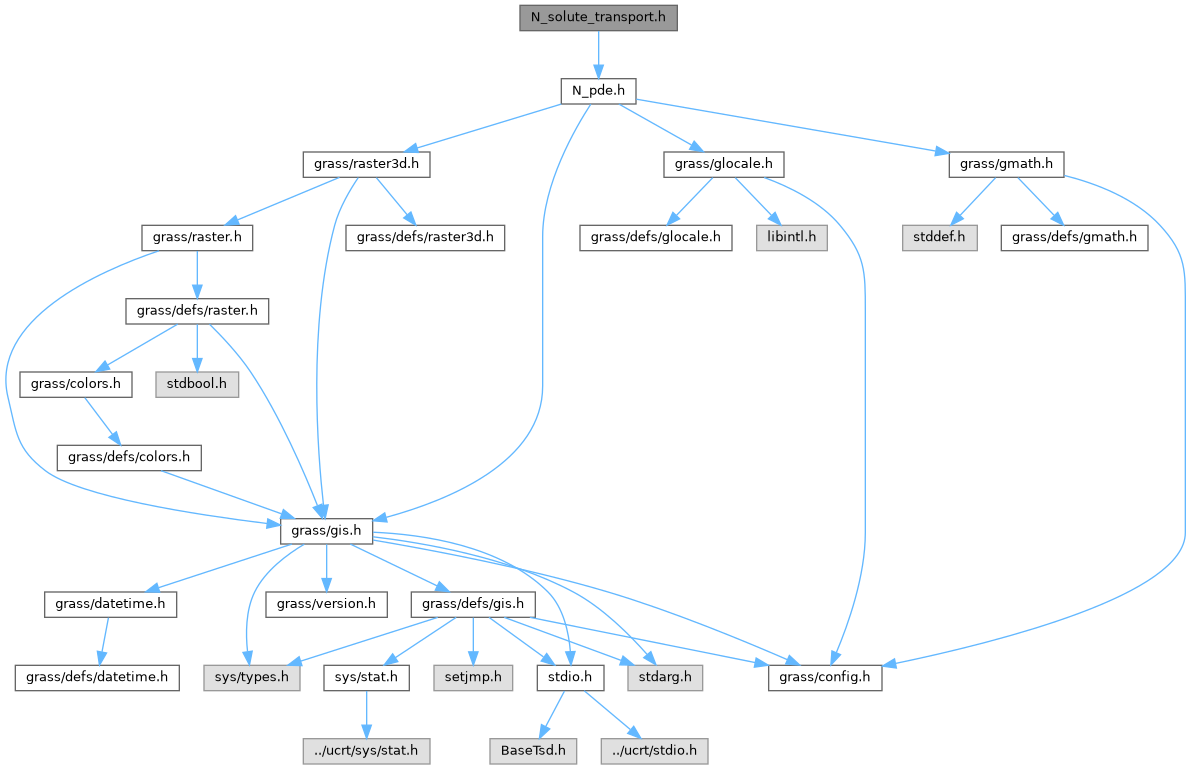GRASS GIS 8 Programmer's Manual  8.2.2dev(2023)-5c00b4e944
N_solute_transport.h File Reference
#include "N_pde.h"
Include dependency graph for N_solute_transport.h:Go to the source code of this file.

## Data Structures

struct  N_solute_transport_data3d

struct  N_solute_transport_data2d

## Functions

N_data_starN_callback_solute_transport_3d (void *solutedata, N_geom_data *geom, int col, int row, int depth)
This is just a placeholder. More...

N_data_starN_callback_solute_transport_2d (void *solutedata, N_geom_data *geom, int col, int row)
This callback function creates the mass balance of a 5 point star. More...

N_solute_transport_data3dN_alloc_solute_transport_data3d (int cols, int rows, int depths)
Alllocate memory for the solute transport data structure in three dimensions. More...

N_solute_transport_data2dN_alloc_solute_transport_data2d (int cols, int rows)
Alllocate memory for the solute transport data structure in two dimensions. More...

void N_free_solute_transport_data3d (N_solute_transport_data3d *data)
Release the memory of the solute transport data structure in three dimensions. More...

void N_free_solute_transport_data2d (N_solute_transport_data2d *data)
Release the memory of the solute transport data structure in two dimensions. More...

void N_calc_solute_transport_disptensor_2d (N_solute_transport_data2d *data)
Compute the dispersivity tensor based on the solute transport data in 2d. More...

void N_calc_solute_transport_disptensor_3d (N_solute_transport_data3d *data)
Compute the dispersivity tensor based on the solute transport data in 3d. More...

void N_calc_solute_transport_transmission_2d (N_solute_transport_data2d *data)
Compute the transmission boundary condition in 2d. More...

void N_calc_solute_transport_transmission_3d (N_solute_transport_data3d *data)

## ◆ N_alloc_solute_transport_data2d()

 N_solute_transport_data2d* N_alloc_solute_transport_data2d ( int cols, int rows )

Alllocate memory for the solute transport data structure in two dimensions.

The solute transport data structure will be allocated including all appendant 2d arrays. The offset for the 2d arrays is one to establish homogeneous Neumann boundary conditions at the calculation area border. This data structure is used to create a linear equation system based on the computation of solute transport in porous media with the finite volume method.

Parameters
 cols int rows int
Returns
N_solute_transport_data2d *

Definition at line 457 of file n_solute_transport.c.

## ◆ N_alloc_solute_transport_data3d()

 N_solute_transport_data3d* N_alloc_solute_transport_data3d ( int cols, int rows, int depths )

Alllocate memory for the solute transport data structure in three dimensions.

The solute transport data structure will be allocated including all appendant 3d arrays. The offset for the 3d arrays is one to establish homogeneous Neumann boundary conditions at the calculation area border. This data structure is used to create a linear equation system based on the computation of solute transport in porous media with the finite volume method.

Parameters
 cols int rows int depths int
Returns
N_solute_transport_data3d *

Definition at line 402 of file n_solute_transport.c.

## ◆ N_calc_solute_transport_disptensor_2d()

 void N_calc_solute_transport_disptensor_2d ( N_solute_transport_data2d * data )

Compute the dispersivity tensor based on the solute transport data in 2d.

The dispersivity tensor is stored in the data structure. To compute the dispersivity tensor, the dispersivity lentghs and the gradient field must be present.

This is just a simple tensor computation which should be extended.

Todo:
Change the tensor calculation to a mor realistic algorithm
Parameters
Returns
void *

Definition at line 651 of file n_solute_transport.c.

## ◆ N_calc_solute_transport_disptensor_3d()

 void N_calc_solute_transport_disptensor_3d ( N_solute_transport_data3d * data )

Compute the dispersivity tensor based on the solute transport data in 3d.

The dispersivity tensor is stored in the data structure. To compute the dispersivity tensor, the dispersivity lentghs and the gradient field must be present.

This is just a simple tensor computation which should be extended.

Todo:
Change the tensor calculation to a mor realistic algorithm
Parameters
Returns
void *

Definition at line 710 of file n_solute_transport.c.

## ◆ N_calc_solute_transport_transmission_2d()

 void N_calc_solute_transport_transmission_2d ( N_solute_transport_data2d * data )

Compute the transmission boundary condition in 2d.

This function calculates the transmission boundary condition for each cell with status N_CELL_TRANSMISSION. The surrounding gradient field is used to verfiy the flow direction. If a flow goes into a cell, the concentration (data->c) from the neighbour cell is added to the transmission cell. If the flow from several neighbour cells goes into the cell, the concentration mean is calculated.

The new concentrations are written into the data->c_start array, so they can be handled by the matrix assembling function.

Parameters
Returns
void *

Definition at line 578 of file n_solute_transport.c.

## ◆ N_calc_solute_transport_transmission_3d()

 void N_calc_solute_transport_transmission_3d ( N_solute_transport_data3d * data )

## ◆ N_callback_solute_transport_2d()

 N_data_star* N_callback_solute_transport_2d ( void * solutedata, N_geom_data * geom, int col, int row )

This callback function creates the mass balance of a 5 point star.

The mass balance is based on the common solute transport equation:This equation is discretizised with the finite volume method in two dimensions.

Parameters
 solutedata * N_solute_transport_data2d - a void pointer to the data structure geom N_geom_data * col int row int
Returns
N_data_star * - a five point data star

Definition at line 184 of file n_solute_transport.c.

References N, NE, NW, SE, SW, and W.

## ◆ N_callback_solute_transport_3d()

 N_data_star* N_callback_solute_transport_3d ( void * solutedata, N_geom_data * geom, int col, int row, int depth )

This is just a placeholder.

Definition at line 28 of file n_solute_transport.c.

## ◆ N_free_solute_transport_data2d()

 void N_free_solute_transport_data2d ( N_solute_transport_data2d * data )

Release the memory of the solute transport data structure in two dimensions.

Parameters
Returns
void *

Definition at line 536 of file n_solute_transport.c.

## ◆ N_free_solute_transport_data3d()

 void N_free_solute_transport_data3d ( N_solute_transport_data3d * data )

Release the memory of the solute transport data structure in three dimensions.

Parameters
Returns
void *

Definition at line 499 of file n_solute_transport.c.